﻿ 基于光滑模型约束的同震滑动分布正则化反演 Regularized Inversion of Coseismic Slip Distribution Based on Smoothness-Constrained Model

Vol.07 No.05(2017), Article ID:22456,10 pages
10.12677/AG.2017.75066

Regularized Inversion of Coseismic Slip Distribution Based on Smoothness-Constrained Model

Xiaozhong Tong1,2, Dongjun Cheng1*, Siyang Wu1, Jie Dong1, Yujun Guo1

1School of Geosciences and Info-Physics, Central South University, Changsha Hunan

2School of Earth and Ocean Sciences, University of Victoria, Victoria, Canada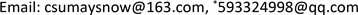Received: Oct. 3rd, 2017; accepted: Oct. 8th, 2017; published: Oct. 26th, 2017ABSTRACT

Estimating the spatial distribution of coseismic slip is an ill-posed inverse problem, and the solution is non-unique. In order to obtain stable solution for coseismic slip inversion, regularization method with smoothness-constrained was imposed. For the implementation of inverse algorithm, we construct a smoothness-constraint model with non-uniform slip on the fault plane, and propose a fast and stable method for choosing regularization parameter. In order to get reasonable coseismic slip distribution, non-negative least squares method is adopted. Inversion for a synthetic model with uniform coseismic slip distribution shows that the inverse algorithm is effective and stable, and non-negative least squares method can reconstruct reasonable results. We conduct inversions on the 2005 Nias earthquake with smoothness-constraint regularized method, and make a comparison of other results. The results for the 2005 Nias earthquake indicate the maximum slip is about 12.8 m, which agrees well spatially with the coseismic slip distribution of Konca. The released moment based on the estimated coseismic slip distribution is 9.91 × 1012 Nm, which is equivalent to a moment magnitude (Mw) of 8.6 and almost identical to the value determined by USGS. The inversion results for synthetic coseismic slip distribution model and real earthquakes show that the smoothness-constrained regularized inversion method is effective, and can be reasonable to reconstruct coseismic slip distribution on the fault plane.

Keywords:Coseismic Slip Distribution, Regularized Inversion, Smoothness-Constrained Model, Non-Negative Least Squares, The 2005 Nias Earthquake

1中南大学地球科学与信息物理学院，湖南 长沙

2维多利亚大学，地球与海洋科学学院，加拿大 维多利亚1. 引言

2. 模型与算法

2.1. 正则化方法

${P}^{\beta }\left(m\right)={\varphi }_{d}+\beta {\varphi }_{m}$ (1)

${P}^{\beta }\left(m\right)={‖{W}_{d}\left(Gm-{d}^{obs}\right)‖}^{2}+\beta {‖{W}_{m}m‖}^{2}$ (2)

$\left({G}^{\text{T}}{W}_{d}^{\text{T}}{W}_{d}G+\beta {W}_{m}^{\text{T}}{W}_{m}\right)\cdot m={G}^{\text{T}}{W}_{d}^{\text{T}}{W}_{d}{d}^{obs}$ (3)

${m}_{rec}={\left({G}^{\text{T}}{W}_{d}^{\text{T}}{W}_{d}G+\beta {W}_{m}^{\text{T}}{W}_{m}\right)}^{-1}\cdot {G}^{\text{T}}{W}_{d}^{\text{T}}{W}_{d}{d}^{obs}$ (4)

2.2. 光滑约束模型构建

${\nabla }^{2}s\approx \frac{\frac{{s}_{i+1,j}-{s}_{i,j}}{\Delta {x}_{2}}-\frac{{s}_{i,j}-{s}_{i-1,j}}{\Delta {x}_{1}}}{\frac{\Delta {x}_{1}+\Delta {x}_{2}}{2}}+\frac{\frac{{s}_{i,j+1}-{s}_{i,j}}{\Delta {y}_{2}}-\frac{{s}_{i,j}-{s}_{i,j-1}}{\Delta {y}_{1}}}{\frac{\Delta {y}_{1}+\Delta {y}_{2}}{2}}$ (5)

$\left\{\begin{array}{l}diag\left(D,0\right)=-2\left(\frac{1}{\Delta {x}_{1}\Delta {x}_{2}}+\frac{1}{\Delta {y}_{1}\Delta {y}_{2}}\right)\\ diag\left(D,+1\right)=\frac{2}{\Delta {y}_{2}\left(\Delta {y}_{1}+\Delta {y}_{2}\right)}\\ diag\left(D,-1\right)=\frac{2}{\Delta {y}_{1}\left(\Delta {y}_{1}+\Delta {y}_{2}\right)}\\ diag\left(D,+\text{nc}\right)=\frac{2}{\Delta {x}_{2}\left(\Delta {x}_{1}+\Delta {x}_{2}\right)}\\ diag\left(D,-\text{nc}\right)=\frac{2}{\Delta {x}_{1}\left(\Delta {x}_{1}+\Delta {x}_{2}\right)}\end{array}$ (6)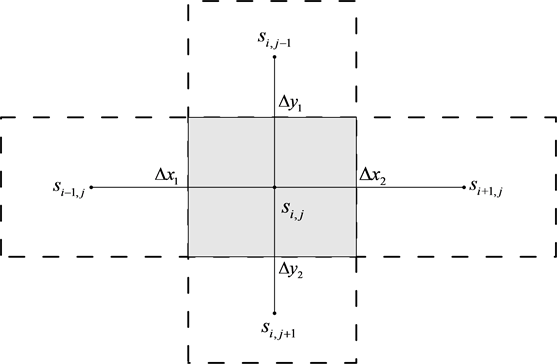Figure 1. Schematic figure of fault patches for the smoothed coseismic slip

2.3. 正则化因子求取

${\beta }_{\mathrm{min}}=\underset{1\le i\le N}{\mathrm{Min}}|\sum _{j=1}^{M}{a}_{mj}|$ (7)

${\beta }_{\mathrm{max}}=\underset{1\le i\le N}{\mathrm{Max}}|\sum _{j=1}^{M}{a}_{mj}|$ (8)

${\beta }_{best}=\underset{{\beta }_{\mathrm{min}}\le \beta \le {\beta }_{\mathrm{max}}}{\mathrm{max}}\left\{\frac{{\zeta }^{\prime }{\eta }^{″}-{\zeta }^{″}{\eta }^{\prime }}{{\left[{\left({\zeta }^{\prime }\right)}^{2}+{\left({\eta }^{\prime }\right)}^{2}\right]}^{3/2}}\right\}$ (9)

3. 理论模型反演试算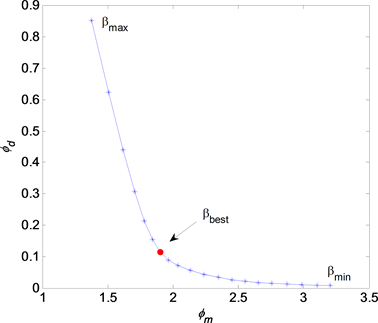Figure 2. The best regularization parameter used in the inversion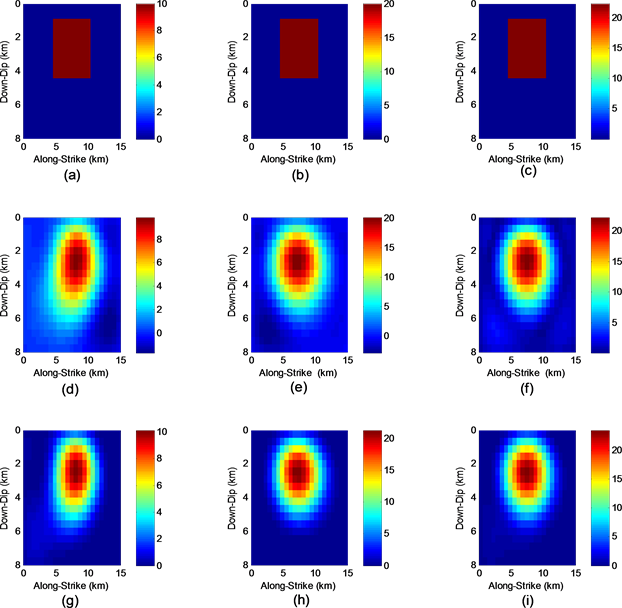Figure 3. Coseismic slip distribution of a synthetic model and inversion results

4. 2005年Nias地震

Nias地震是2005年3月28日发生在印度尼西亚的尼亚斯岛附近海域的8.6级强烈地震，其震中位置为(2.074˚N, 97.013˚E)，震源深度为30 km。该地震同震形变观测数据选用Kreemer等提供的解算结果  ，表2给出了10个GPS观测站点的三维同震位移。通过数据可以看出，地表水平移动的最大值约4.5 m，平均下沉的最大值约2.9 m。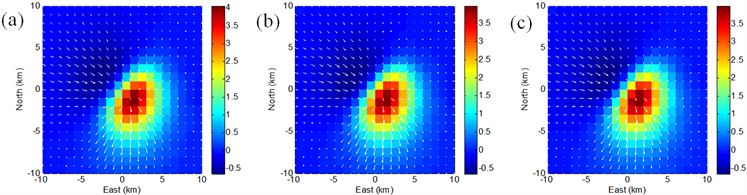Figure 4. Coseismic displacement of a synthetic model and inversion results; (a)Observed data; (b)LS inversion; (c)NNLS inversionTable 1. Numerical experiments with regularized inversion for noise dataTable 2. Coseismic displacement of GPS sites for Nias earthquake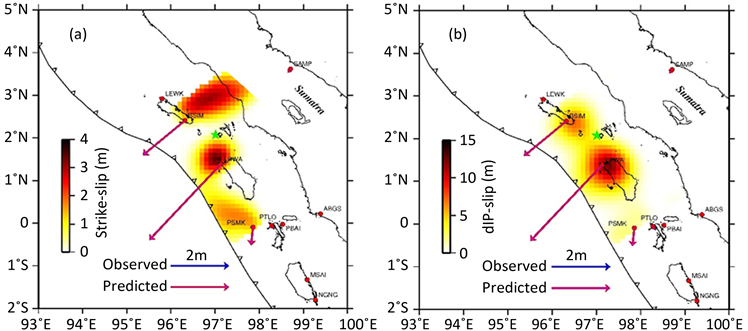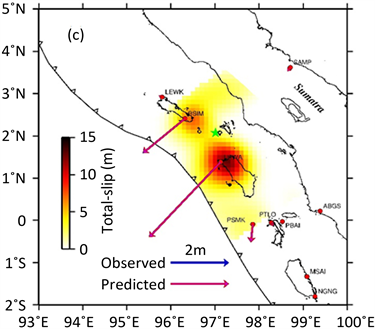Figure 5. Coseismic slip distribution of the 2005 Nias earthquake; (a) Strike-slip distribution; (b) Dip-slip distribution; (c) Total-slip distribution

5. 结论与讨论

Regularized Inversion of Coseismic Slip Distribution Based on Smoothness-Constrained Model[J]. 地球科学前沿, 2017, 07(05): 653-662. http://dx.doi.org/10.12677/AG.2017.75066

1. 1. Masterlark, T. (2003) Finite Element Model Predictions of Static Deformation from Dislocation Source in a Subduction Zone: Sensi-tivities to Homogeneous, Isotropic, Poisson-Solid, and Half-Space. Journal of Geophysical Research, 108, 2540. https://doi.org/10.1029/2002JB002296

2. 2. Tong, X.P., Sandwell, D.T. and Fialko, Y. (2010) Coseismic Slip Model of the 2008 Wenchuan Earthquake Derived from Joint Inversion of Interferometric Synthetic Aperture Radar, GPS, and Field Data. Journal of Geophysical Research, 115, Article ID: B04314. https://doi.org/10.1029/2009JB006625

3. 3. Maerten, F., Resor, P., Pollard, D., et al. (2005) Inverting for Slip on Three-Dimensional Fault Surfaces Using Angular Dislocations. Bulletin of the Seismological Society of America, 95, 1654-1665. https://doi.org/10.1785/0120030181

4. 4. Barnhart, W.D. and Lohman, R.B. (2010) Automated Fault Model Discretization for Inversions for Coseismic Slip Distributions. Journal of Geophysical Research, 115, Article ID: B10419. https://doi.org/10.1029/2010JB007545

5. 5. Pollitz, F.F., Burgmann, R. and Banerjee, P. (2011) Geodetic Slip Model of the 2011 M9.0 Tohoku Earthquake. Geophysical Research Letters, 38, Article ID: L00G08. https://doi.org/10.1029/2011GL048632

6. 6. Fukuda, J. and Johnson, K.M. (2010) Mix Linear-Non-Linear Inversion of Crustal Deformation Data: Bayesian Inference of Model, Weighting and Regularization Parameters. Geophysical Journal International, 181, 1441-1458.

7. 7. Ziv, A., Doin, M.P. and Grandin, R. (2013) What Can Be Learned from Underdetermined Geodetic Slip Inversions: The Parkfield GPS Network Example. Geophysical Journal International, 194, 1900-1908. https://doi.org/10.1093/gji/ggt207

8. 8. Yabuki, T. and Matsu’ura, M. (1992) Geodetic Data Inversion Using a Bayesian Infor-mation Criterion for Spatial Distribution of Fault Slip. Geophysical Journal International, 109, 363-375. https://doi.org/10.1111/j.1365-246X.1992.tb00102.x

9. 9. Fukuda, J. and Johnson, K.M. (2008) A Fully Bayesian Inversion for Spatial Distribution of Fault Slip with Objective Smoothing. Bulletin of the Seismological Society of America, 98, 1128-1146. https://doi.org/10.1785/0120070194

10. 10. Hreinsdóttir, S., Freymueller, J.T., Fletcher, H.J., et al. (2003) Coseismic Slip Distri-bution of the 2002 Mw7.9 Denali Fault Earthquake, Alaska, Determined from GPS Measurements. Geophysical Research Letters, 30, 1670. https://doi.org/10.1029/2003GL017447

11. 11. Jiang, G.Y., Xu, C.J. and Wen, Y.M. (2013) Inversion for Coseismic Slip Distri-bution of the 2010 Mw6.9 Yushu Earthquake from InSAR Data using Angular Dislocations. Geophysical Journal International, 181, 1441-1458.

12. 12. 许才军, 邓长勇, 周力璇. 利用方差分量估计的地震同震滑动分布反演[J]. 武汉大学学报信息科学版, 2016, 41(1): 37-44.

13. 13. Okada, Y. (1985) Surface Deformation Due to Shear and Ensile Faults in a Half-Space. Bulletin of the Seismo-logical Society of America, 75, 1135-1154.

14. 14. Okada, Y. (1992) Internal Deformation Due to Shear and Ensile Faults in a Half-Space. Bulletin of the Seismological Society of America, 82, 1018-1040.

15. 15. Tikhonov, A.N. and Arsenin, V.Y. (1997) Solution of Ill-Posed Problems. John Wiley, New York.

16. 16. Lawson, C.L. and Hanson, R.J. (1995) Solving Least Squares Problems. Society for Industrial and Applied Mathematics, Philadephia.

17. 17. Bro, R. and de Jong, S. (1997) A Fast Non-Negativity-Constrained Least Squares Algorithm. Journal of Chemometrics, 11, 393-401. https://doi.org/10.1002/(SICI)1099-128X(199709/10)11:5<393::AID-CEM483>3.0.CO;2-L

18. 18. Symithe, S.J., Calais, E., Haase, J.S., et al. (2013) Coseismic Slip Distribution of the 2010 M7.0 Haiti Earthquake and Resulting Stress Changes on Regional Faults. Bulletin of the Seismological Society of America, 103, 2326-2343. https://doi.org/10.1785/0120120306

19. 19. Farquharson, C.G. and Oldenburg, D.W. (2004) A Comparison of Automatic Tech-niques for Estimating the Regularization Parameter in Non-Linear Inverse Problems. Geophysical Journal International, 156, 411-425. https://doi.org/10.1111/j.1365-246X.2004.02190.x

20. 20. 陈小斌, 赵国泽, 汤吉, 等. 大地电磁自适应正则化反演算法[J]. 地球物理学报, 2005, 48(4): 937-946.

21. 21. 刘海飞, 阮百尧, 柳建新, 等. 混合范数下的最优化反演方法[J]. 地球物理学报, 2007, 50(6): 1877-1883.

22. 22. Kreemer, C., Blewitt, G. and Maerten, F. (2005) Co- and Postseismic Deformation of the 28 March 2005 Nias Mw8.7 Earthquake from Continuous GPS Data. Geophysical Research Letters, 33, L07307.

23. 23. Konca, A.O., Hiorleifsdottir, V., Song, T.A., et al. (2007) Rupture Kinematics of the 2005, Mw 8.6, Nias-Simeulue Earthquake from the Joint Inversion of Seismic and Geodetic Data. Bulletin of the Seismological Society of America, 97, 307-322. https://doi.org/10.1785/0120050632